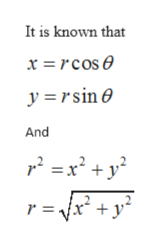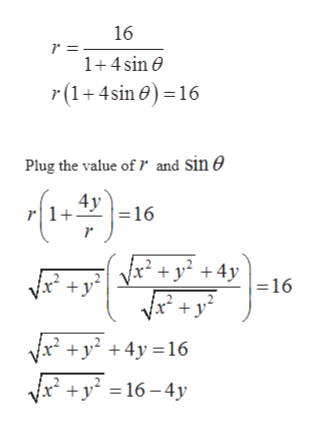# Convert the polar equation to rectangular coordinates. (Use variables x and y as needed.) r = 16/1 + 4 sin(θ)

Question
19 views

Convert the polar equation to rectangular coordinates. (Use variables x and y as needed.)
r = 16/1 + 4 sin(θ)

check_circle

Step 1

Given that

Step 2

To convert the polar equation to rectangular coordinatehelp_outlineImage TranscriptioncloseIt is known that x =rcose y rsine And = x2 + y2 r = fullscreen
Step 3

From equatio...help_outlineImage Transcriptionclose16 1+4sin e r(1 4sin)16 and sin e Plug the value of 4y r1+ 16 +y 16 Vx2 y 4y16 Vx2 16-4y fullscreen

### Want to see the full answer?

See Solution

#### Want to see this answer and more?

Solutions are written by subject experts who are available 24/7. Questions are typically answered within 1 hour.*

See Solution
*Response times may vary by subject and question.
Tagged in

### Functions